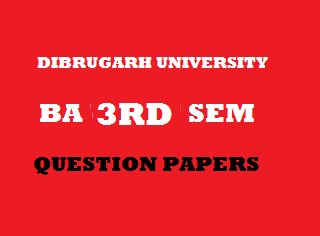## Wednesday, January 02, 20192012
(November)
EDUCATION
(General)
Course: 301
(Measurement and Evaluation In Education)
Full Marks: 80
Pass Marks: 32
Time: 3 hours
The figures in the margin indicate full marks for the questions

1. Choose the correct answer from the given responses/Answer the following: 1x8=8
1. Evaluation of all the aspects of an individual is called
1. Continuous evaluation.
2. Comprehensive evaluation.
3. Formative evaluation.
4. None of the above.
1. The degree to which a test measures what it purports to measure is called
1. Reliability of the test.
2. Validity of the test.
3. Objectivity of the test.
4. Norms of the test.
1. If the IQ of a 15-year-old student becomes 120, his mental age will be
1. 13 years.
2. 15 years.
3. 18 years.
4. 20 years.
1. The number of cards in Rorschach inkblot test is
1. 10.
2. 12.
3. 20.
4. 30.
1. The total number of questions of Binet-Simon scale of 1908 was
1. 55.
2. 59.
3. 65.
4. 54.
1. The total subtest of differential aptitude test (DAT) was
1. 6.
2. 12.
3. 10.
4. 8.

1. The mean and median of a set of scores are found to be 50 and 55 respectively. Determine the mode of the set of scores.
2. Calculate the mean of the following set of scores:
10,  8, 12,  6, 4, 2
2. Write short notes on: 4x5=20
1. Concept of continuous and comprehensive evaluation.
2. Predictive validity.
3. Objective-type examination.
4. Nonverbal intelligence.
5. Skewness.
3. What do you mean by measurement? What are different types of measurement? Mention the difference between physical measurement and psychological measurement. 1+3+7=11
Or
Explain the scope and needs of measurement and evaluation in education. 5+6=11
4. What is meant by reliability of a test? Discuss about the test-retest and split-half technique of estimating reliability of a test. 1+3+3=7
Or
What is meant by educational achievement? Discuss the advantages and disadvantages of essay-type question. 1+3+3=7
5. Discuss about Stanford revision of Binet-Simon scale of 1916. 8
Or
What is meant by personality? What are different methods of personality assessment? Discuss briefly.        1+7=8
6. What is meant by the term ‘statistics’? Explain its need and importance in the field of education.           2+5=7
7. Calculate quartile deviation from the frequency distribution table:
 Class Interval Frequency 50 – 5445 – 4940 – 4435 – 3930 – 3425 – 2920 – 2415 – 1910 – 14 35810149632 N = 60

8. Draw a histogram from the following frequency distribution table: 4

 Class Interval Frequency 20 – 2425 – 2930 – 3435 – 3940 – 4445 – 4950 – 5455 – 5960 – 6465 – 69 238101696432 N = 63

9. Determine the correlation coefficient by rank difference method from the following two sets of scores and interpret the result: 7+1=8
 X: 19 29 24 27 16 56 22 32 18 24 Y: 32 24 42 54 42 67 58 57 49 87

***